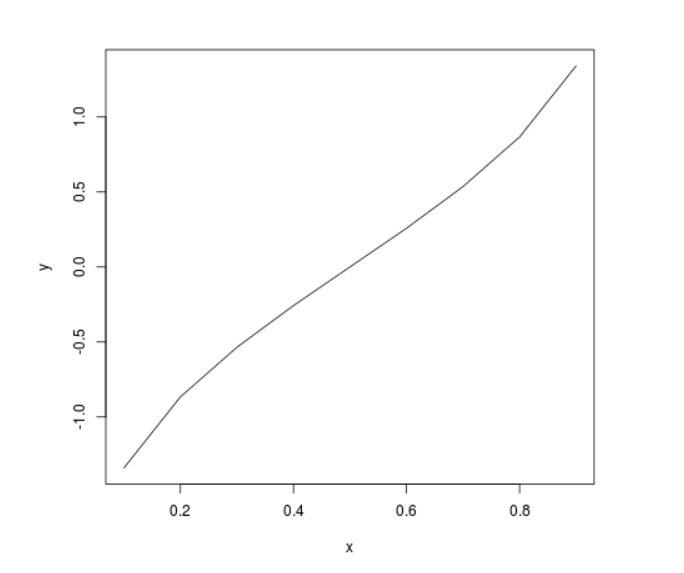# Perform the Inverse Probability Cumulative Density Analysis on t-Distribution in R Programming – qt() Function

qt() function in R Language is used to return the inverse probability cumulative density of the Student t-distribution.

Syntax: qt(x, df)
Parameters:
x: Random variable
df: Degree of Freedom

Example 1:

## Python3

 `# R Program to perform Inverse` `# Cumulative Density Analysis`   `# Calling qt() Function` `qt(.``95``, ``10``)` `qt(.``542``, ``15``)`

Output:

``` 1.812461
 0.1072654```

Example 2:

## Python3

 `# R Program to perform Inverse` `# Cumulative Density Analysis`   `# Creating a vector` `x <``-` `seq(.``1``, .``9``, by ``=` `.``1``)`   `# Calling qt() Function` `y <``-` `qt(x, ``15``)`   `# Plotting probability distribution graph` `plot(x, y, ``type` `=``"l"``)`

Output:Whether you're preparing for your first job interview or aiming to upskill in this ever-evolving tech landscape, GeeksforGeeks Courses are your key to success. We provide top-quality content at affordable prices, all geared towards accelerating your growth in a time-bound manner. Join the millions we've already empowered, and we're here to do the same for you. Don't miss out - check it out now!

Previous
Next